# Texas Go Math Kindergarten Lesson 12.4 Answer Key Subtraction Word Problems

Refer to our Texas Go Math Kindergarten Answer Key Pdf to score good marks in the exams. Test yourself by practicing the problems from Texas Go Math Kindergarten Lesson 12.4 Answer Key Subtraction Word Problems.

## Texas Go Math Kindergarten Lesson 12.4 Answer Key Subtraction Word Problems

Unlock the Problem

DIRECTIONS: Listen to and act out the subtraction word problem. Write the numbers and trace the symbols to complete the subtraction sentence.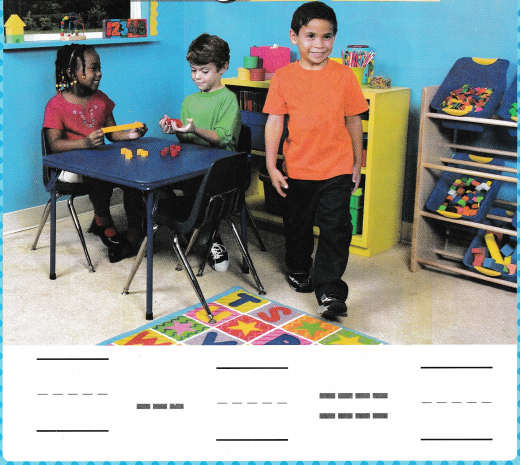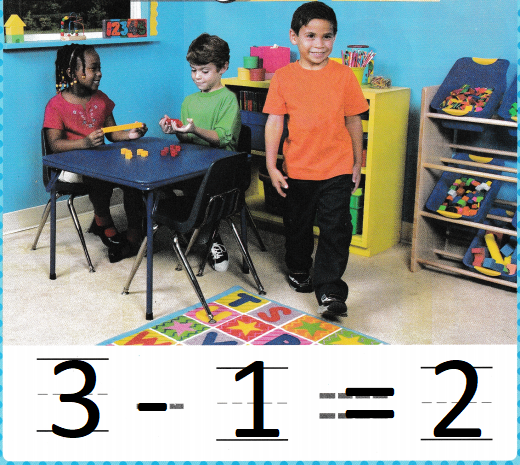Try Another Problem

DIRECTIONS: 1. Listen to and use cubes to act out the subtraction word problem. Write the numbers and trace the symbols to complete the subtraction sentence.

Question 1.Explanation:
2 children playing with toys
1 child left
2 – 1 = 1
so, 1 child playing with toys.

Share and Show

DIRECTIONS: 2. Listen to and use cubes to act out the subtraction word problem. Write and trace to complete the subtraction sentence.

Question 2.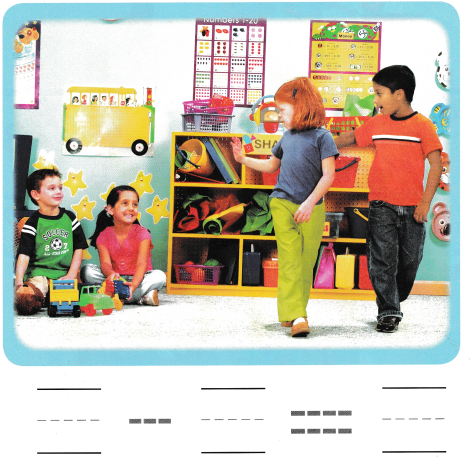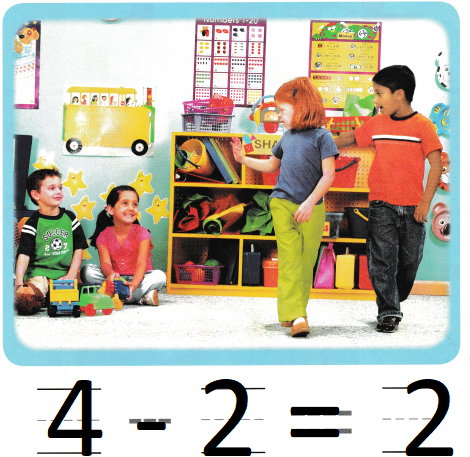Explanation:
4 kids playing with toys
2 kids left
4 – 2 = 2
so, 2 kids are remaining

HOME ACTIVITY • Tell your child a short subtraction word problem. Have him or her use objects to act out the word problem.Explanation:
There are 5 trucks
2 driven away
5 – 3 = 2
so, 3 trucks are remaining.

DIRECTIONS: Choose the correct answer. 3. There are three children in a group. One child walks away. Use cubes to act out 3 – 1. How many children are left? 4. There are five children. Then two children leave. Act out 5 – 2. How many children are left? 5. There are four children. One child rolls away on skates. Act it out. What does the picture show?

Question 3.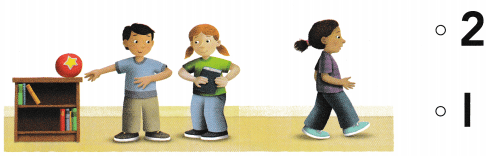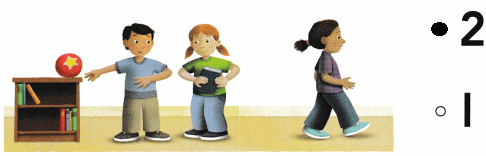Explanation:
There are three children in a group.
One child walks away.
3 – 1 = 2
so, 2 children are left.

Question 4.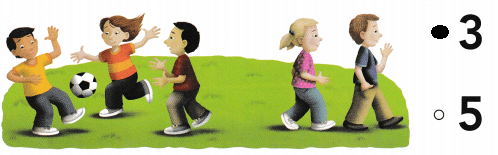Explanation:
There are five children.
Then two children leave
5 – 2 = 3
so, 3 children are still playing.

Question 5.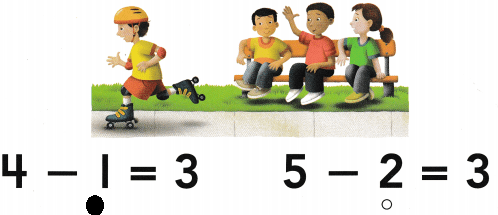Explanation:
There are four children.
One child rolls away on skates.
4 – 1 = 3
3 children are sitting on the bench.

### Texas Go Math Kindergarten Lesson 12.4 Homework and Practice Answer Key

DIRECTIONS: 1. There are 3 puppies in the basket. Juan takes one puppy out of the basket. How many puppies are left in the basket? Write the numbers and trace the symbols to complete the subtraction sentence.

Question 1.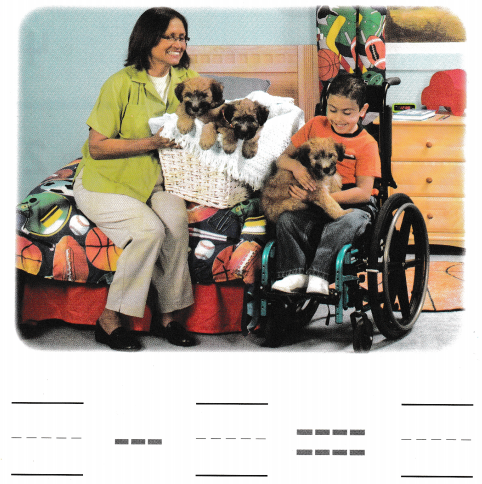Explanation:
There are 3 puppies in the basket.
Juan takes one puppy out of the basket.
3 – 1 = 2
so, 2 puppies are in the basket.

DIRECTIONS: Choose the correct answer. 2. There are five balloons. Four balloons fly away. How many balloons are left? 3. There are four soccer balls. One soccer ball rolls away. How many soccer balls are left? 4. There are two frogs. One frog hops away. How many frogs are left?

Lesson Check

Question 2.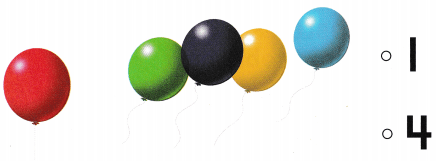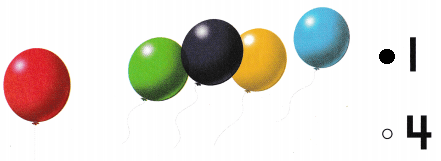Explanation:
There are five balloons.
Four balloons fly away.
5 – 4 = 1
so, 1 balloon is left

Question 3.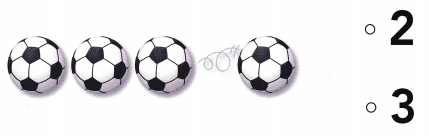Explanation:
There are four soccer balls.
One soccer ball rolls away.
4 – 1 = 3
so, 3 soccer balls are left

Question 4.